##1. Chasles-Steiner definition of a conic

The Chasles-Steiner definition of a conic ([ChaslesConiques, p.5], [Baker, p. 73]), illustrated by the figure below, can be described as follows.
Consider the two pencils A* and B* of lines through points A and B correspondingly and a homographic relation L'=F(L) between the lines of the two pencils.
The Chasles-Steiner method of definition describes a conic as the geometric locus of intersection-points P of lines L and L'.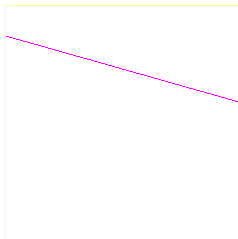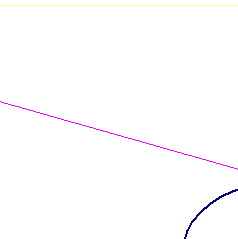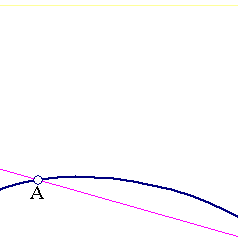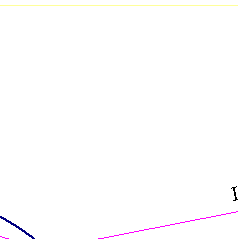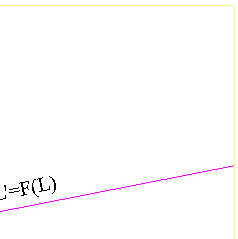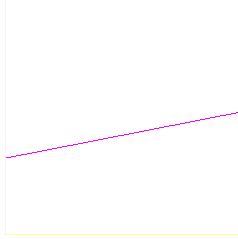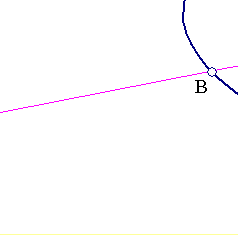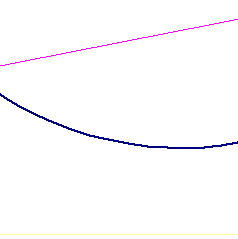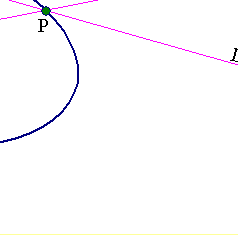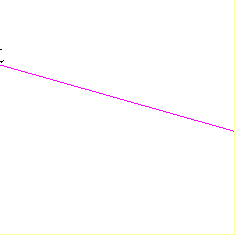##2. Pencil parameterization

The pencil A* of all lines through point A can be parameterized by the points of an arbitrary but fixed (projective) line E. To every line L through Acorresponds the intersection point Q of L with E. Introducing (projective) coordinates for the line E we can correspond to L a definite number x. Various such coordinate systems, corresponding to different positions of the parameterizing line E, are related by homographic relations x'=(ax+b)/(cx+d) (see HomographicRelation.html ).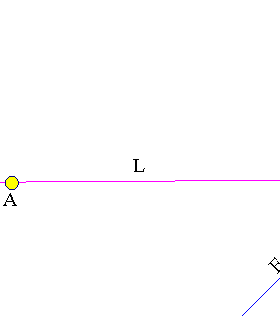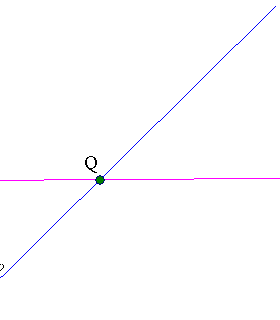##3. A proof of the claim

To prove the claim of section-1, on the generation of conics, I use a projective coordinate system, namely barycentric coordinates (see BarycentricCoordinates.html ) with respect to a triangle with vertices {A,B,C}. Point C is taken to be one of the points of the locus i.e one of the intersection points of line L with line F(L). The pencils are parameterized with the aid of a line E passing through C.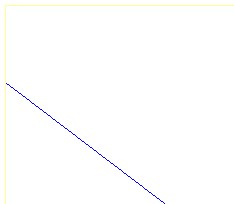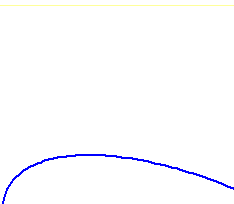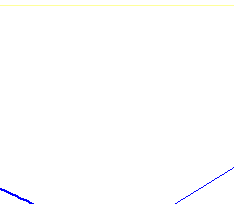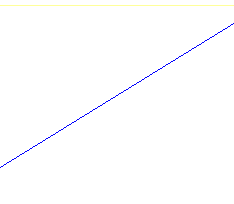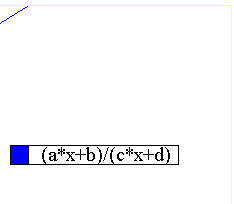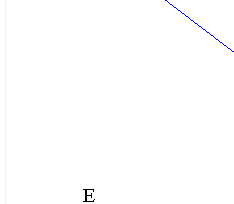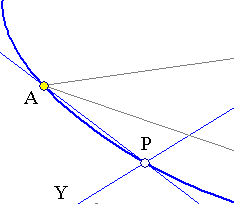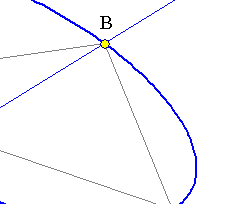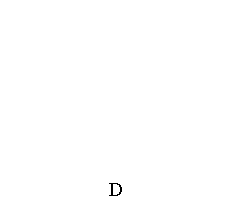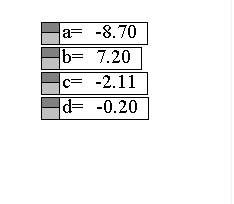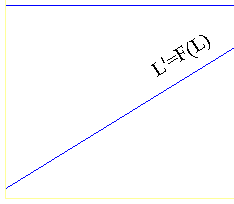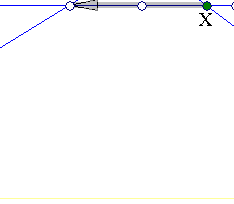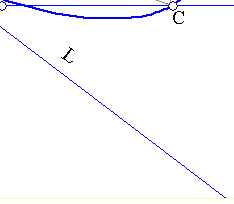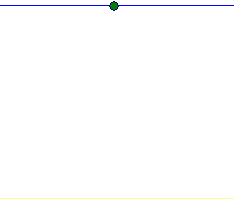In such a coordinate system points of the line E are represented as C+tD and the homographic relation is given by a function f(t)=(at+b)/(ct+d).
Since C is mapped to itself f(0)=0 i.e. b=0. D is a fixed point on line E. Writing D in the form D=pA+qB+rC we have for X the projective coordinates (tp,tq,1+tr) and for Y=F(X) the coordinates (f(t)p,f(t)q,1+f(t)r). Line L has coefficients given by the vector product (1,0,0)x(tp,tq,1+tr)=(0,-1-tr,tq).
Line L' has coefficients given by the vector product (0,1,0)x(f(t)p,f(t)q,1+f(t)r)=(1+f(t)r,0,-f(t)p).
Their intersection point is given by the vector product (0,-1-tr,tq) x (1+f(t)r,0,-f(t)p) = ((1+tr)f(t)p, tq(1+f(t)r), (1+tr)(1+f(t)r)).
Equating this vector with (kx,ky,kz) and eliminating {t,k} we find after a short calculation
(c+r(a-d))xy + (-ap)yz + (dq)zx =0.
This is the equation of a conic passing through the three base points {A,B,C} of the coordinate system. See the file ChaslesSteinerExample.html for another example where a similar calculation is carried out giving a characteristic property of hyperbolas. See also the file Maclaurin_Chasles_Steiner.html for anexploration of the relation of this theorem to the earlier one by Maclaurin (see Maclaurin.html ).

##4. The degenerate case

Without loss of generality we may assume that point D coincides with the intersection of the parameterizing line E with line AB. From the expressionD = pA+qB+rC, this is equivalent with the condition r = 0 and implies the simpler form of the equation of the conic
(c)xy + (-ap)yz + (dq)zx = 0.
The determinant of the corresponding matrix representing the conic in these coordinates (up to the factor -2) is  Det = cadpq.
The conic is degenerate (product of two lines) if and only if Det = 0, thus giving the condition  cadpq = 0. Here {p,q} are different from zero, since otherwiseD would coincide with A or B. Hence the condition is equivalent with  cad = 0.  Again {a,d} are different from zero, since otherwise the homographic relation would be a constant one. Thus Det = 0 is equivalent with c = 0 and the matrix equation reduces to
z(dqx - apy) = 0.
which expresses indeed the product of the two lines
z = 0  and   (dq)x - (ap)y = 0.
The first line coincides with AB and the second passes through C and the point on the line AB:  D' = (ap)A + (dq)B. Thus we come at the result
If the Chasles-Steiner generation produces a degenerate conic then one of the lines is the line through the poles (A,B) of the procedure.

Remark A criterion of degeneration is the discovery of three collinear points lying on the locus described by the Chasles-Steiner procedure. This remark has many applications to particular configurations. An example is given in the next section. See also Chasles_Steiner_Line_Locus.html .

##5.  Line locus

Given a triangle ABC a line p and two points {B', C'} on side BC consider all points P on line p. Let {Bx, Cy} denote the intersection points of lines {B'P, C'P} with sides{AB, AC} respectively. Assume that {x,y} denote the coordinates of these points for systems of line coordinates along {BA, CA} starting respectively at {B,C}. Then these coordinates satisfy a relation of the form y = ax/(bx+c).
The inverse is also valid, i.e. if on sides {BA, CA} points {Bx, Cy} are taken, in such a way that their coordinates {x,y} satisfy the above relation, then lines {B'Bx, C'Cy}intersect at a point P describing a line p, as x varies.

The proof of the property follows by composing two homographic relations describing the perspectivities through points {B',C'}. The first corresponds to Bx of line AB the point point P on line p. The second corresponds to P on lin p point Cy. The composition of the two is the correspondence Bx --> By described by the composition of two Moebius transformations which is also a Moebius transformation, thereby proving the claim (see HomographicRelation.html , HomographicRelationExample.html ).
The inverse follows from the previous section. According to it if {Bx, Cy} are related by such a relation, then the intersection point of lines {B'Bx, C'Cy} describes aconic which passes through the points {B',C'}. But the conic passes also through the limit point F on BC resulting for x-->0 (can be computed analytically see calculation below).  Hence the conic contains a line (BC) and must be degenerate, thus, P describes a second line passing through F.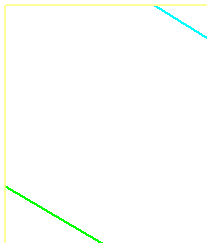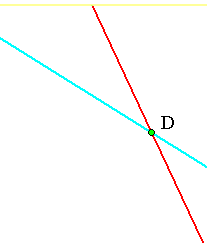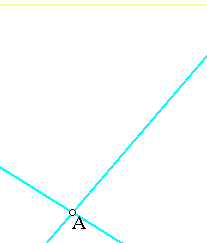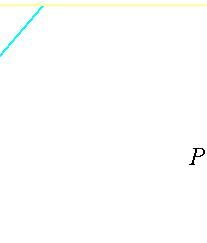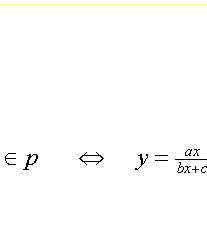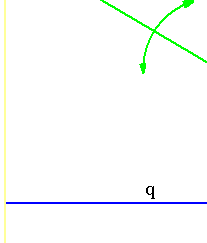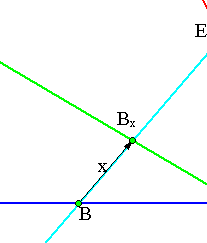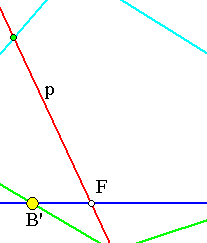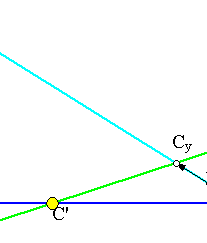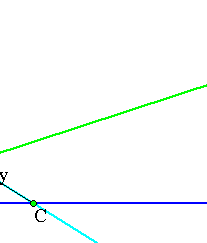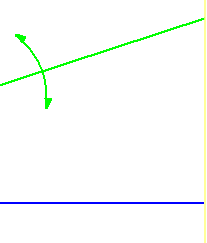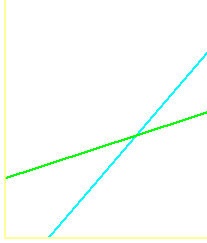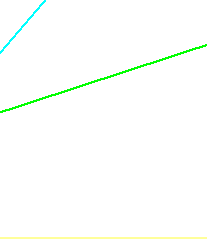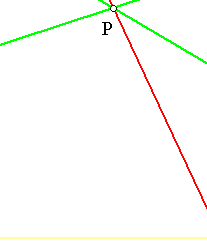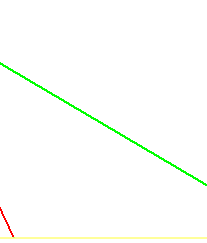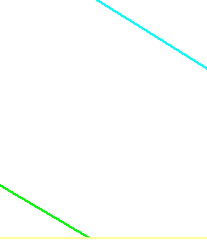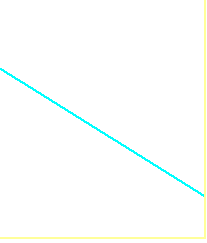To find the limit position F of P for x-->0 project P on BC to F' (not drawn). Then  PB' = PF'/sin(B'), PC' = PF'/sin(C'). From triangle BB'Bx it is(sine-theorem) (x/sin(B')) = BB'/sin(Bx) => sin(B') = (x/BB')sin(Bx). Analogous expression for sin(C') gives PB'/PC' = sin(C')/sin(B') =((y/CC')sin(Cx))/((x/BB')sin(Bx)) and since the limit y/x for x-->0 is (a/c) the above equality gives that FB'/FC' = (a/c)(BB'/CC')(sin(C)/sin(B)).

HomographicRelation.html
BarycentricCoordinates.html
ChaslesSteinerExample.html
Maclaurin_Chasles_Steiner.html
Maclaurin.html
Chasles_Steiner_Line_Locus.html
HomographicRelationExample.html

### Bibliography

[Baker] Baker, H. F. Plane Geometry New York, Chelsea Publishing Company 1971
[ChaslesConiques] Chasles, M. Traite des sections coniques Gauthier-Villars, Paris, 1865